# How to Find the DC Gain of a Transfer Function (Examples Included)

Contents

## What is a Transfer Function?

A transfer function describes the relationship between the output signal of a control system and the input signal. A block diagram is a visualization of the control system that uses blocks to represent the transfer function and arrows representing the different input and output signals.

The transfer function is a convenient representation of a linear time-invariant dynamical system. Mathematically the transfer function is a function of complex variables

For any control system, there is a reference input known as excitation or cause that operates through a transfer function to produce an effect resulting in a controlled output or response.

Thus, the cause and effect relationship between output and input is linked to each other through a transfer function. In a Laplace Transform, if the input is represented by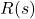and the output is represented by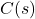.

The control system transfer function is defined as the Laplace transform ratio of the output variable to the Laplace transform of the input variable, assuming that all initial conditions are zero.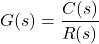## What is DC Gain?

The transfer function has many useful physical interpretations. The steady-state gain of a system is simply the ratio of the output and the input in steady-state represented by a real number between negative infinity and positive infinity.

When a stable control system is stimulated with a step input, the response at a steady-state reaches a constant level.

The term DC gain is described as the ratio of amplitude between the response of the steady-state and the step input.

DC gain is the ratio of the magnitude of the response to the steady-state step to the magnitude of the step input. The final value theorem demonstrates that DC gain is the value of the transfer function assessed at 0 for stable transfer functions.

## Time Response of First Order Systems

The order of a dynamic system is the order of the highest derivative of its governing differential equation. First-order systems are the simplest dynamic systems to analyze.

To understand the concept of steady-state gain or DC gain, consider a general first-order transfer function.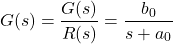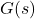can also be written as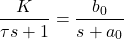Here,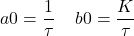is called the time constant. K is called the DC gain or steady-state gain

## How to Find the DC Gain of a Transfer Function

DC gain is the ratio of the steady-state output of a system to its constant input, i.e., steady-state of the unit step response.

To find the DC gain of a transfer function, let us consider both continuous and discrete Linear Transform Inverse (LTI) systems.

Continuous LTI system is given as

(1)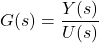Discrete LTI system is given as

(2)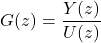Use final value theorem to compute the steady-state of the unit step response.

(3)(4)(5)is stable and all poles lie on the left hand side

Hence,

(6)The formula of the final value theorem used for a continuous LTI system is

(7)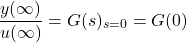The formula of the final value theorem used for a discrete LTI system is

(8)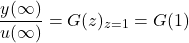In both cases, if the system has an integration the result will be.

The DC gain is the ratio between the steady-state input and the steady-state derivative of the output can be obtained via differentiation of the obtained output. It is nearly same for both continuous and discrete system.

### Differentiation in the Continuous Domain

In the continuous system or ‘s’ domain, the equation (1) is differentiated by multiplying the equation by ‘s’.

(9)where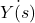is the Laplace transform of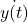### Differentiation in the Discrete Domain

Th derivative in the discrete domain can be obtained by a first difference.

(10)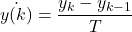(11)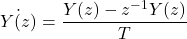(12)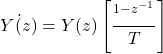(13)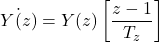Thus to differentiate in the discrete domain, we need to multiply### Numerical Examples To Find DC Gain

#### Example 1

Consider the continuous transfer function,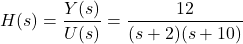To find the DC gain (steady-state gain) of the above transfer function, apply the final value theorem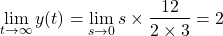Now the DC gain is defined as the ratio of steady state value to the applied unit step input.

DC Gain =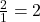Hence it is important to note that the concept of DC Gain is applicable only to those systems which are stable in nature.

#### Example 2

Determine the DC gain for the equationThe step response of the above transfer equation is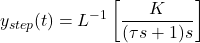Now, apply the final value theorem to find the DC gain.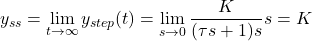Want To Learn Faster? 🎓
Get electrical articles delivered to your inbox every week.
No credit card required—it’s 100% free.